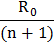# To verify Ohm’s law, a student is provided with a test resistor, a high resistance, a small resistance, two identical galvanometersandand a variable voltage source V. the correct circuit to carry out the experiment is a)b)c)d)## Question ID - 150246 :- To verify Ohm’s law, a student is provided with a test resistor, a high resistance, a small resistance, two identical galvanometersandand a variable voltage source V. the correct circuit to carry out the experiment is a)b)c)d)3537

 To decrease the range of an ammeter, its resistance need to be increased. An ammeter has resistanceand range I. Which of the following resistance can be connected in series with it to decreases its range to? a)b)c)d) None of these